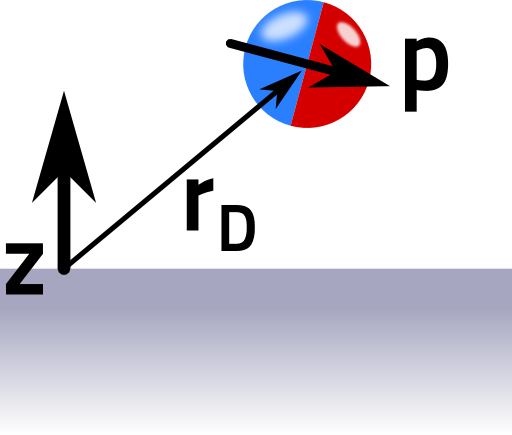## A Dipole in front of a flat Metallic surfaceThe Green's function can be used to find the electric field or electrostatic potential for arbitrary charge distributions. You will learn that the polarization of a dipole in front of a flat metallic surface enormously affects the overall field.

## Problem StatementUse the Green's function to calculate the electrostatic potential $$\phi\left(\mathbf{r}\right)$$  for a dipole $$\mathbf{p}$$ at $$\mathbf{r}_{D}$$ in front of a flat metallic surface terminated at $$z=0$$. Determine  the main contributions to the potential for $$\left|\mathbf{r}_{D}\right|\ll\left|\mathbf{r}\right|$$. Which has a stronger fall-of: $$x$$- or $$z$$-polarization? Can you explain this in simple words?

## Hints

What is the Green's function $$G\left(\mathbf{r},\mathbf{r}^{\prime}\right)$$ of a flat metallic surface? Remember the mirror charge technique (grounded metallic corner problem) and that the Green's function is basically just the field of a point charge at $$\mathbf{r}^{\prime}$$. Then, $$\phi\left(\mathbf{r}\right)=\int G\left(\mathbf{r},\mathbf{r}^{\prime}\right)\rho\left(\mathbf{r}^{\prime}\right)dV^{\prime}$$.

The charge distribution of a mathematical dipole is formally given by $$\rho_{D}\left(\mathbf{r}\right)=-\mathbf{p}\cdot\nabla\delta\left(\mathbf{r}-\mathbf{r}_{D}\right)$$. A Taylor expansion can be a quite useful tool for approximations and also outlined in the dipole calculations.

## The Electrostatic Potential

Let us write down the Green's function for the given system. Of course we know that for a single charge at $\mathbf{r}^{\prime} = \left(\begin{array}{c}x^{\prime}\\y^{\prime}\\z^{\prime}\end{array}\right)\ ,$the mirror charge would have to be placed at $\mathbf{r}_{m}^{\prime} = \left(\begin{array}{c}x^{\prime}\\y^{\prime}\\{\color{red}-}z^{\prime}\end{array}\right)\ .$ Then, the Green's function is given by $G\left(\mathbf{r},\mathbf{r}^{\prime}\right) = \frac{1}{4\pi\varepsilon_{0}}\left(\frac{1}{\left|\mathbf{r}-\mathbf{r}^{\prime}\right|}-\frac{1}{\left|\mathbf{r}-\mathbf{r}_{m}^{\prime}\right|}\right)\ .$To find the electrostatic potential for the dipole, we have to use the charge distribution of it:$\rho_{D}\left(\mathbf{r}\right) = -\mathbf{p}\cdot\nabla\delta\left(\mathbf{r}-\mathbf{r}_{D}\right)\ .$We obtained this formula in "The Electric Field of a Dipole" , if you want to check the expression. So, we use the solution of the Poisson equation to formally find$\begin{eqnarray*} \phi\left(\mathbf{r}\right)&=&\int G\left(\mathbf{r},\mathbf{r}^{\prime}\right)\rho\left(\mathbf{r}^{\prime}\right)dV^{\prime}\\&=&-\mathbf{p}\cdot\int G\left(\mathbf{r},\mathbf{r}^{\prime}\right)\nabla_{\mathbf{r}^{\prime}}\delta\left(\mathbf{r}^{\prime}-\mathbf{r}_{D}\right)dV^{\prime}\\&=&\mathbf{p}\cdot\int\left[\nabla_{\mathbf{r}^{\prime}}G\left(\mathbf{r},\mathbf{r}^{\prime}\right)\right]\delta\left(\mathbf{r}^{\prime}-\mathbf{r}_{D}\right)dV^{\prime} \end{eqnarray*}$by partial integration. We now have to determine the gradient of the Green's function with respect to its second variable! First for the $$x$$ component:$\begin{eqnarray*} \frac{\partial}{\partial x^{\prime}}G\left(\mathbf{r},\mathbf{r}^{\prime}\right)&=&\frac{1}{4\pi\varepsilon_{0}}\frac{\partial}{\partial x^{\prime}}\left(\frac{1}{\left|\mathbf{r}-\mathbf{r}^{\prime}\right|}-\frac{1}{\left|\mathbf{r}-\mathbf{r}_{m}^{\prime}\right|}\right)\\&=&\frac{1}{4\pi\varepsilon_{0}}\left(\frac{x-x^{\prime}}{\left|\mathbf{r}-\mathbf{r}^{\prime}\right|^{3}}-\frac{x-x^{\prime}}{\left|\mathbf{r}-\mathbf{r}_{m}^{\prime}\right|^{3}}\right) \end{eqnarray*}$and likewise for the $$y$$ component. Note that we have used $$\left|\mathbf{r}-\mathbf{r}^{\prime}\right|=\sqrt{\left(x-x^{\prime}\right)^{2}+\left(y-y^{\prime}\right)^{2}+\left(z-z^{\prime}\right)^{2}}$$ and that we have differentiated with respect to the second variable of the Green's function! Now for the $$z$$ component:$\frac{\partial}{\partial z^{\prime}}G\left(\mathbf{r},\mathbf{r}^{\prime}\right) = \frac{1}{4\pi\varepsilon_{0}}\left(\frac{z-z^{\prime}}{\left|\mathbf{r}-\mathbf{r}^{\prime}\right|^{3}}{\color{red}+}\frac{z-z^{\prime}}{\left|\mathbf{r}-\mathbf{r}_{m}^{\prime}\right|^{3}}\right)\ .$We can already see the difference between both components of the Green's function's gradient: a sign change (denoted red). Now we put these components of the gradient into the solution of the Poisson equation and perform the integration:$\begin{eqnarray*} \phi\left(\mathbf{r}\right)&=&\mathbf{p}\cdot\int\left[\nabla_{\mathbf{r}^{\prime}}G\left(\mathbf{r},\mathbf{r}^{\prime}\right)\right]\delta\left(\mathbf{r}^{\prime}-\mathbf{r}_{D}\right)dV^{\prime}\\&=&\frac{1}{4\pi\varepsilon_{0}}\mathbf{p}\cdot\left(\begin{array}{c}\left(x-x_{D}\right)\left[\left|\mathbf{r}-\mathbf{r}_{D}\right|^{-3}-\left|\mathbf{r}-\mathbf{r}_{mD}\right|^{-3}\right]\\\left(y-y_{D}\right)\left[\left|\mathbf{r}-\mathbf{r}_{D}\right|^{-3}-\left|\mathbf{r}-\mathbf{r}_{mD}\right|^{-3}\right]\\\left(z-z_{D}^{\prime}\right)\left[\left|\mathbf{r}-\mathbf{r}_{D}\right|^{-3}+\left|\mathbf{r}-\mathbf{r}_{mD}\right|^{-3}\right]\end{array}\right)\ \text{with}\\\mathbf{r}_{mD}&=&\left(\begin{array}{c}x_{D}\\y_{D}\\{\color{red}-}z_{D}\end{array}\right)\ . \end{eqnarray*}$This is the solution of the electrostatic potential of a dipole in front of a flat metallic surface. We can directly see that it corresponds to a two-dipole-solution: a mirror-dipole appears at $$\mathbf{r}_{mD}$$ (see figure). Now let us try to understand the potential a little further

## Taylor Expansion of the Potential

From a heuristic point of view it is clear that the dipole in $$z$$ direction causes a mirror dipole pointing in the same direction. The $$x$$-polarization, parallel to the surface, corresponds to dipoles with opposite direction. Hence, the $$z$$-polarization should correspond to an overall dipolar field and the $$x$$ polarization to a quadrupolar one. This is what we should find out.Far away from the sources for $$\left|\mathbf{r}_{D}\right|\ll\left|\mathbf{r}\right|$$, we may Taylor-expand the denominators in the potential. The terms look rather similar, so we just have to calculate:$\frac{1}{\left|\mathbf{r}-\mathbf{r}_{D}\right|^{3}} \approx \frac{1}{\left|\mathbf{r}\right|^{3}}+\frac{3\mathbf{r}\cdot\mathbf{r}_{D}}{\left|\mathbf{r}\right|^{5}}$ and analog for $$\mathbf{r}_{mD}$$, where the $$z$$ component switches the sign. This is the important difference and we find$\begin{eqnarray*} \frac{1}{\left|\mathbf{r}-\mathbf{r}_{D}\right|^{3}}-\frac{1}{\left|\mathbf{r}-\mathbf{r}_{mD}\right|^{3}}&\approx&\frac{6z\, z_{D}}{\left|\mathbf{r}\right|^{5}}\ \text{and}\\\frac{1}{\left|\mathbf{r}-\mathbf{r}_{D}\right|^{3}}+\frac{1}{\left|\mathbf{r}-\mathbf{r}_{mD}\right|^{3}}&\approx&\frac{2}{\left|\mathbf{r}\right|^{3}}+\frac{6\left(x\, x_{D}+y\, y_{D}\right)}{\left|\mathbf{r}\right|^{5}}\ .\end{eqnarray*}$In this far-field approximation, the potential reads as$\phi\left(\mathbf{r}\right) \approx \frac{1}{4\pi\varepsilon_{0}}\mathbf{p}\cdot\left(\begin{array}{c}6x\, z\, z_{D}/\left|\mathbf{r}\right|^{5}\\6y\, z\, z_{D}/\left|\mathbf{r}\right|^{5}\\2z/\left|\mathbf{r}\right|^{3}+6z\left(x\, x_{D}+y\, y_{D}\right)/\left|\mathbf{r}\right|^{5}\end{array}\right)$ and we have shown that the potential has a stronger fall-off behaviour for the $$x$$ and $$y$$ polarizations which goes effectively like $$\propto Q_{ij}x^{i}x^{j}/r^{5}$$ whereas the $$z$$ polarization behaves as $$\propto p_{z}z/r^{3}$$. These potentials correspond to the quadrupolar and dipolar fields. We will find out later that oscillating dipoles radiate much faster than quadrupoles. This is the reason why a dipole polarized parallel to the surface will basically heat the metal whereas the perpendicula polarization has a chance to escape the system. The polarization has thus a dramatic effect on the effectivity of emitter/metal-plate-systems.

Check again the multipole moment section for more information on multipole moments.

## Background: Dipole Emitters in Front of Flat Metallic Surfaces

In this problem, we discuss the electrostatic dipole in the vicinity of a flat metal which is of huge interest both in applied and fundamental physics: think of a dipole antenna with a metal backplate or an emitting molecule. Of course such systems demand an understanding of full electrodynamics. The point is, however, that the main features will remain; $$x$$- and $$z$$-polarization will radiate differently.

It gets even more interesting if we do not have a perfect metal. Then, the electric field can penetrate the conductor and a closely placed dipole can excite surface waves as predicted by Sommerfeld more than one hundred years ago. Even though this effect was not the explanation for long-range radio transmissions, the theory of Sommerfeld is still at the core of plasmonics, the field that deals with these so-called surface plasmon polaritons. For much more information on dipoles in front of flat metallic surfaces please have a look at Lukas Novotny's freely available book chapter on the topic.